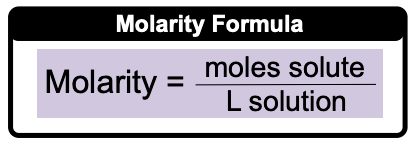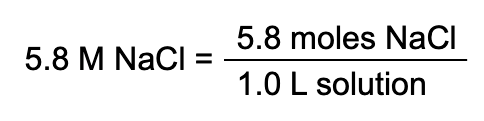Clutch Prep is now a part of Pearson

# Molarity

See all sections
Sections
Solutions
Solubility and Intermolecular Forces
Solutions: Mass Percent
Percent Concentrations
Molarity
Osmolarity
Parts per Million (ppm)
Solubility: Temperature Effect
Intro to Henry's Law
Henry's Law Calculations
Dilutions
Solution Stoichiometry
Electrolytes (Simplified)
Equivalents
Molality
The Colligative Properties
Boiling Point Elevation
Freezing Point Depression
Osmosis
Osmotic Pressure

Ever wonder how a mass amount such as moles can be converted into the volume amount of liters? Well, molarity serves as the bridge between moles and liters.

###### Molarity

Concept #1: Calculate MolarityExample #1: Calculate the molarity of a solution prepared by dissolving 23.7 g NaOH in enough water to make 2.50 L of solution.

Concept #2: Using Molarity to Calculate UnknownsExample #2: How many grams of Na3PO4 (MW: 163.94 g/mol) are present in 300.0 mL of a 0.550 M Na3POsolution?

Practice: What volume in (µL) of 0.125 M HBr contains 0.170 moles HBr?

Practice: Hypernatremia is a medical condition where a patient has high levels of sodium in their blood, and is the result of the body containing too little water. A patient has a measured sodium level of 165 mM. If 30.0 mL of their blood were drawn, what mass (in ng) of sodium would be present?

Practice: 2.64 grams of an unknown compound was dissolved in water to yield 150 mL of solution. The concentration of the solution was 0.075 M. What was the molecular weight of the substance?

Practice: A solution with a final volume of 750.0 mL was prepared by dissolving 30.00 mL of benzene (C6H6, density = 0.8787 g/mL) in dichloromethane. Calculate the molarity of benzene in the solution.